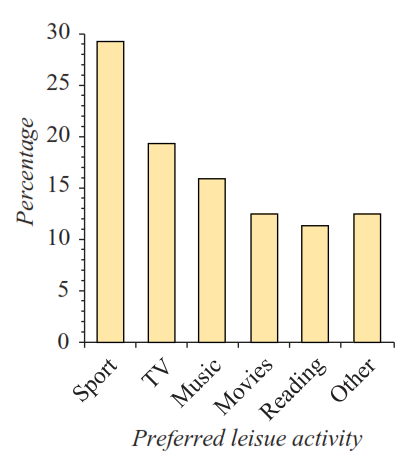# 2118 Univariate Data - Bar Charts

Approved & Edited by ProProfs Editorial Team
The editorial team at ProProfs Quizzes consists of a select group of subject experts, trivia writers, and quiz masters who have authored over 10,000 quizzes taken by more than 100 million users. This team includes our in-house seasoned quiz moderators and subject matter experts. Our editorial experts, spread across the world, are rigorously trained using our comprehensive guidelines to ensure that you receive the highest quality quizzes.
| By Anthony Nunan
A
Anthony Nunan
Community Contributor
Quizzes Created: 159 | Total Attempts: 46,613
Questions: 18 | Attempts: 666SettingsBar Charts, Stacked or Segmented Bar Charts

• 1.

### The image above is a :

• A.

Percent frequency bar chart

• B.

Segmented bar chart

• C.

Histogram

• D.

Frequency bar chart

• E.

I don't know

A. Percent frequency bar chart
Explanation
The image above is a percent frequency bar chart because it displays the frequency of different categories as a percentage of the total. Each bar represents a category, and the height of the bar represents the percentage of occurrences of that category. This type of chart is useful for comparing the relative frequencies of different categories and identifying any patterns or trends.

Rate this question:

• 2.

### The image above is a :

• A.

I don't know

• B.

Percent frequency bar chart

• C.

Segmented bar chart

• D.

Histogram

• E.

Frequency bar chart

B. Percent frequency bar chart
Explanation
The image above is a percent frequency bar chart because it represents the distribution of a variable as a percentage of the total. Each bar in the chart represents a category, and the height of the bar represents the percentage of data points that fall into that category. This type of chart is useful for comparing the relative frequencies of different categories and understanding the overall distribution of the data.

Rate this question:

• 3.

### The image above is a :

• A.

Frequency bar chart

• B.

Segmented bar chart

• C.

Histogram

• D.

Percent frequency bar chart

• E.

I don't know

A. Frequency bar chart
Explanation
The image above is a frequency bar chart because it displays the frequency or count of different categories or values on the y-axis, while the x-axis represents the categories or values themselves. Each bar represents a category or value, and its height corresponds to the frequency or count. This type of chart is commonly used to show the distribution or frequency of data in different categories.

Rate this question:

• 4.

### The image above is a :

• A.

I don't know

• B.

Frequency bar chart

• C.

Segmented bar chart

• D.

Histogram

• E.

Percent frequency bar chart

B. Frequency bar chart
Explanation
The image above is a frequency bar chart because it displays the frequency or count of different categories or values on the y-axis, while the x-axis represents the categories or values themselves. Each bar represents a category or value, and its height corresponds to its frequency or count. This type of chart is commonly used to show the distribution or frequency of data in a visual format.

Rate this question:

• 5.

### The image above is a :

• A.

Frequency bar chart

• B.

Segmented bar chart

• C.

Histogram

• D.

Percent frequency bar chart

• E.

I don't know

C. Histogram
Explanation
Histograms are numerical data and the columns are joined

Rate this question:

• 6.

### The image above is a :

• A.

Frequency bar chart

• B.

Segmented bar chart

• C.

Histogram

• D.

Percent frequency bar chart

• E.

I don't know